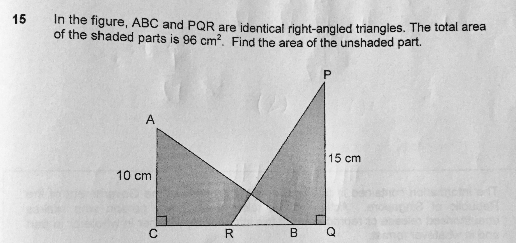# QuestionAnyone help me with attached question

Thanks

If they are identical triangle, and they share the same overlapped unshaded area, that means the shaded portion of each triangle is equal.

Each shaded portion of one triangle is 96/2= 48cmsq

Area of one triangle= 1/2 * 10 *15= 75 cmsq

Therefore unshaded region = 75 – 48= 27 cmsq.

0 Replies 2 Likes ✔Accepted Answer

As the right-angled triangles are identical,, area of unshaded part ——- [(1/2) x 15 x 10 x 2 – 96]/2 = 27

Ans : 27 sq cm

0 Replies 1 Like